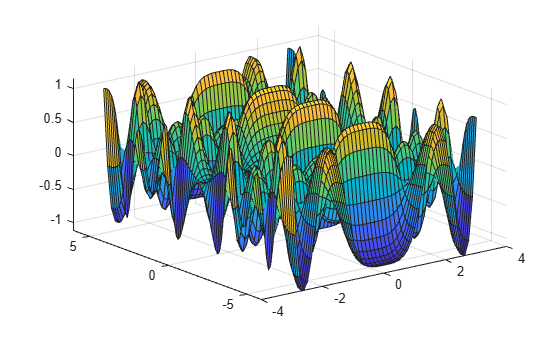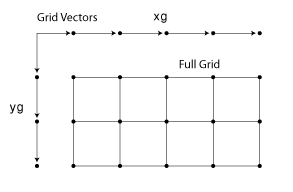ndgrid

Rectangular grid in N-D space

Description

example

[X1,X2,...,Xn] = ndgrid(x1,x2,...,xn) replicates the grid vectors x1,x2,...,xn to produce an n-dimensional full grid.

example

[X1,X2,...,Xn] = ndgrid(xg) specifies a single grid vector xg to use for all dimensions. The number of output arguments you specify determines the dimensionality n of the output.

Examples

collapse all

Create a 2-D grid from the vectors [1 3 5 7 9 11 13 15 17 19] and [2 4 6 8 10 12].

[X,Y] = ndgrid(1:2:19,2:2:12)
X = 10×6

1     1     1     1     1     1
3     3     3     3     3     3
5     5     5     5     5     5
7     7     7     7     7     7
9     9     9     9     9     9
11    11    11    11    11    11
13    13    13    13    13    13
15    15    15    15    15    15
17    17    17    17    17    17
19    19    19    19    19    19

Y = 10×6

2     4     6     8    10    12
2     4     6     8    10    12
2     4     6     8    10    12
2     4     6     8    10    12
2     4     6     8    10    12
2     4     6     8    10    12
2     4     6     8    10    12
2     4     6     8    10    12
2     4     6     8    10    12
2     4     6     8    10    12

Evaluate and plot the function

${x}_{1}{e}^{-{x}_{1}^{2}-{x}_{2}^{2}}$

over the gridded domain

$-2<{x}_{1}<2$ and $-2<{x}_{2}<2$.

Create a grid of values for the domain.

[X1,X2] = ndgrid(-2:.2:2);

Evaluate the function over the domain.

Z = X1 .* exp(-X1.^2 - X2.^2);

Generate a mesh plot of the function.

mesh(X1,X2,Z)In R2016b and later releases, this task does not require the use of ndgrid. Instead, you can construct the grid using implicit expansion with these commands:

x = -2:.2:2;

Z1 = x.' .* exp(-(x.').^2 - x.^2);

Create a 2-D grid and calculate some function values on the grid. Interpolate between the assigned values to refine the grid.

Create a coarse grid for $\left(x,y\right)$ in the range $\left[-5,5\right]$.

[X,Y] = ndgrid(-5:0.5:5);

Calculate some function values on the grid and plot the function.

f = sin(X.^2) * cos(Y.^2);
surf(X,Y,f)Interpolate between the points using a more refined grid and plot the result.

[X1,Y1] = ndgrid(-5:0.125:5);
F = interpn(X,Y,f,X1,Y1,'spline');
surf(X1,Y1,F)Input Arguments

collapse all

Grid vectors, specified as vectors containing grid coordinates for each dimension. The grid vectors implicitly define the grid. For example, in 2-D:Data Types: single | double | int8 | int16 | int32 | int64 | uint8 | uint16 | uint32 | uint64
Complex Number Support: Yes

Grid vector for all dimensions, specified as a vector containing grid coordinates. ndgrid uses xg as the grid vector for each dimension.

Data Types: single | double | int8 | int16 | int32 | int64 | uint8 | uint16 | uint32 | uint64
Complex Number Support: Yes

Output Arguments

collapse all

Full grid representation, returned as separate arrays. For each output array Xi, the ith dimension contains copies of the grid vector xi.

collapse all

Convert Between meshgrid and ndgrid Formats

meshgrid and ndgrid create grids using different output formats. Specifically, the first two dimensions of a grid created using one of these functions are swapped when compared to the other grid format. Some MATLAB® functions use grids in meshgrid format, while others use ndgrid format, so it is common to convert grids between the two formats.

You can convert between these grid formats using pagetranspose (as of R2020b) or permute to swap the first two dimensions of the grid arrays. For example, create a 3-D grid with meshgrid.

[X,Y,Z] = meshgrid(1:4,1:3,1:2);

Now transpose the first two dimensions of each grid array to convert the grid to ndgrid format, and compare the results against the outputs from ndgrid.

Xt = pagetranspose(X);
Yt = pagetranspose(Y);
Zt = pagetranspose(Z);
[Xn,Yn,Zn] = ndgrid(1:4,1:3,1:2);
isequal(Xt,Xn) & isequal(Yt,Yn) & isequal(Zt,Zn)
ans =

logical

1

Using pagetranspose is equivalent to permuting the first two dimensions while leaving other dimensions the same. You can also perform this operation using permute(X,[2 1 3:ndims(X)]).